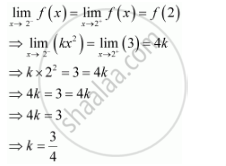Share

# Find the Values Of K So that the Function F Is Continuous at the Indicated Point.F(X) = {(Kxsqrt2, If X<= 2),(3,If X > 2) at X = 2 - CBSE (Commerce) Class 12 - Mathematics

#### Question

Find the values of so that the function f is continuous at the indicated point.

f(x) = {(kx^2, "," if x<= 2),(3, "," if x > 2):} " at x" = 2

#### Solution

The given function is f(x) = {(kx^2, "," if x<= 2),(3, "," if x > 2):}

The given function f is continuous at x = 2, if f is defined at x = 2 and if the value of fat x = 2 equals the limit of f at x = 2

It is evident that is defined at x = 2 and f(2) = k(2)^2 = 4kTherefore, the required value of  k= 3/4

Is there an error in this question or solution?

#### APPEARS IN

NCERT Solution for Mathematics Textbook for Class 12 (2018 to Current)
Chapter 5: Continuity and Differentiability
Q: 27 | Page no. 161

#### Video TutorialsVIEW ALL 

Solution Find the Values Of K So that the Function F Is Continuous at the Indicated Point.F(X) = {(Kxsqrt2, If X<= 2),(3,If X > 2) at X = 2 Concept: Algebra of Continuous Functions.
S